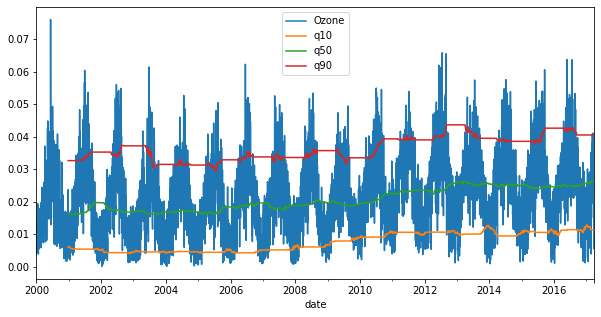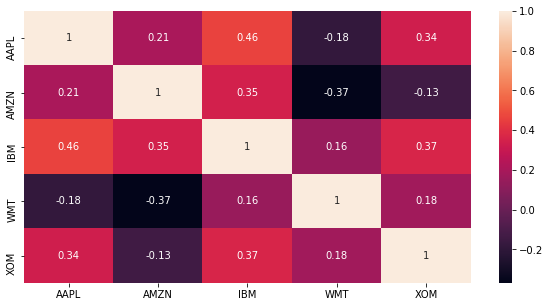import pandas as pd
import numpy as np
import matplotlib.pyplot as plt
import seaborn as sns

plt.rcParams['figure.figsize'] = (10, 5)


## Rolling window function with pandas

• window functions in pandas
• Windows identify sub periods of your time series
• Calculate metrics for sub periods inside the window
• Create a new time series of metrics
• Two types of windows
• Rolling: same size, sliding
• Expanding: Contain all prior values

### Rolling average air quality since 2010 for new york city

The last video was about rolling window functions. To practice this new tool, you'll start with air quality trends for New York City since 2010. In particular, you'll be using the daily Ozone concentration levels provided by the Environmental Protection Agency to calculate & plot the 90 and 360 day rolling average.

data = pd.read_csv('./dataset/ozone_nyc.csv', parse_dates=['date'], index_col='date')
print(data.info())

# Calculate 90d and 360d rolling mean for the last price
data['90D'] = data['Ozone'].rolling('90D').mean()
data['360D'] = data['Ozone'].rolling('360D').mean()

# Plot data
data.loc['2010':].plot(title='New York City');

<class 'pandas.core.frame.DataFrame'>
DatetimeIndex: 6291 entries, 2000-01-01 to 2017-03-31
Data columns (total 1 columns):
#   Column  Non-Null Count  Dtype
---  ------  --------------  -----
0   Ozone   6167 non-null   float64
dtypes: float64(1)
memory usage: 98.3 KB
None### Rolling 360-day median & std. deviation for nyc ozone data since 2000

The last video also showed you how to calculate several rolling statistics using the .agg() method, similar to .groupby().

Let's take a closer look at the air quality history of NYC using the Ozone data you have seen before. The daily data are very volatile, so using a longer term rolling average can help reveal a longer term trend.

You'll be using a 360 day rolling window, and .agg() to calculate the rolling mean and standard deviation for the daily average ozone values since 2000.

data = pd.read_csv('./dataset/ozone_nyc.csv', parse_dates=['date'], index_col='date').dropna()

# Calculate the rolling mean and std here
rolling_stats = data['Ozone'].rolling(360).agg(['mean', 'std'])

# Join rolling_stats with ozone data
stats = data.join(rolling_stats)

# Plot data
stats.plot(subplots=True);### Rolling quantiles for daily air quality in nyc

You learned in the last video how to calculate rolling quantiles to describe changes in the dispersion of a time series over time in a way that is less sensitive to outliers than using the mean and standard deviation.

Let's calculate rolling quantiles - at 10%, 50% (median) and 90% - of the distribution of daily average ozone concentration in NYC using a 360-day rolling window.

data = data.resample('D').interpolate()
print(data.info())

# Create the rolling window
rolling = data['Ozone'].rolling(360)

# Insert the rolling quantiles to the monthly returns
data['q10'] = rolling.quantile(0.1).to_frame('q10')
data['q50'] = rolling.quantile(0.5).to_frame('q50')
data['q90'] = rolling.quantile(0.9).to_frame('q90')

# Plot the data
data.plot()

<class 'pandas.core.frame.DataFrame'>
DatetimeIndex: 6300 entries, 2000-01-01 to 2017-03-31
Freq: D
Data columns (total 1 columns):
#   Column  Non-Null Count  Dtype
---  ------  --------------  -----
0   Ozone   6300 non-null   float64
dtypes: float64(1)
memory usage: 98.4 KB
None

<matplotlib.axes._subplots.AxesSubplot at 0x7f62e2e523d0>## Expanding window functions with pandas

• Expanding windows in pandas
• From rolling to expanding windows
• Calculate metrics for periods up to current date
• New time series reflects all historical values
• Useful for running rate of return, running min/max
• Two options with pandas:
• .expanding()
• .cumsum(),.cumprod(),.cummin(), .cummax()
• How to calculate a running return
• Single period return $r_t$: current prince over last price minus 1: $$r_t = \frac{P_t}{P_{t-1}} - 1$$
• Multi-period return: product of ($1 + r_t$) for all periods, minus 1: $$R_T = (1 + r_1)(1 + r_2) \dots (1 + r_T) - 1$$

### Cumulative sum vs .diff()

In the video, you have learned about expanding windows that allow you to run cumulative calculations.

The cumulative sum method has in fact the opposite effect of the .diff() method that you came across in chapter 1.

To illustrate this, let's use the Google stock price time series, create the differences between prices, and reconstruct the series using the cumulative sum.

data = pd.read_csv('./dataset/google.csv', parse_dates=['Date'], index_col='Date').dropna()

differences = data.diff().dropna()

# Select start price
start_price = data.first('D')

# Calculate cumulative sum
cumulative_sum = start_price.append(differences).cumsum()

# Validate cumulative sum equals data
print(data.equals(cumulative_sum))

True


### Cumulative return on $1,000 invested in google vs apple I To put your new ability to do cumulative return calculations to practical use, let's compare how much$1,000 would be worth if invested in Google ('GOOG') or Apple ('AAPL') in 2010.

data = pd.read_csv('./dataset/apple_google.csv', parse_dates=['Date'], index_col='Date')

investment = 1000

# Calculate the daily returns here
returns = data.pct_change()

# Calculate the cumulative returns here
returns_plus_one = returns + 1
cumulative_return = returns_plus_one.cumprod()

# Calculate and plot the investment return here
cumulative_return.mul(investment).plot();### Annual return correlations among several stocks

You have seen in the video how to calculate correlations, and visualize the result.

In this exercise, we have provided you with the historical stock prices for Apple (AAPL), Amazon (AMZN), IBM (IBM), WalMart (WMT), and Exxon Mobile (XOM) for the last 4,000 trading days from July 2001 until the end of May 2017.

You'll calculate the year-end returns, the pairwise correlations among all stocks, and visualize the result as an annotated heatmap.

data = pd.read_csv('./dataset/5_stocks.csv', parse_dates=['Date'], index_col='Date')

print(data.info())

# Calculate year-end prices here
annual_prices = data.resample('A').last()

# Calculate annual returns here
annual_returns = annual_prices.pct_change()

# Calculate and print the correlation matrix here
correlations = annual_returns.corr()
print(correlations)

# Visualize the correlations as heatmap here
sns.heatmap(correlations, annot=True);
plt.savefig('../images/stock_heatmap.png')

<class 'pandas.core.frame.DataFrame'>
DatetimeIndex: 4001 entries, 2001-07-05 to 2017-05-31
Data columns (total 5 columns):
#   Column  Non-Null Count  Dtype
---  ------  --------------  -----
0   AAPL    4000 non-null   float64
1   AMZN    4000 non-null   float64
2   IBM     4000 non-null   float64
3   WMT     4000 non-null   float64
4   XOM     4000 non-null   float64
dtypes: float64(5)
memory usage: 187.5 KB
None
AAPL      AMZN       IBM       WMT       XOM
AAPL  1.000000  0.208731  0.460568 -0.183553  0.336413
AMZN  0.208731  1.000000  0.346407 -0.367620 -0.133965
IBM   0.460568  0.346407  1.000000  0.155445  0.367253
WMT  -0.183553 -0.367620  0.155445  1.000000  0.178833
XOM   0.336413 -0.133965  0.367253  0.178833  1.000000